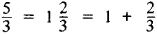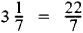# improper fraction

(redirected from improper fractions)
Also found in: Dictionary, Thesaurus.
Related to improper fractions: mixed numbers

## improper fraction

a fraction in which the numerator has a greater absolute value or degree than the denominator, as 7/6 or (x2 + 3)/(x + 1)
Collins Discovery Encyclopedia, 1st edition © HarperCollins Publishers 2005
The following article is from The Great Soviet Encyclopedia (1979). It might be outdated or ideologically biased.

## Improper Fraction

an arithmetic fraction whose numerator is greater than or equal to the denominator—for example, 5/3, 4/2, and 7/7. An improper fraction can be represented, by separating its integral part, in the form of a mixed number—that is, a number having an integral part and a fractional part, for example,Conversely, every mixed number can be written in the form of an improper fraction, for example,## improper fraction

[im′präp·ər ′frak·shən]
(mathematics)
In arithmetic, the quotient of two integers in which the numerator is greater than or equal to the denominator.
In algebra, the quotient of two polynomials in which the degree of the numerator is greater than or equal to that of the denominator.
McGraw-Hill Dictionary of Scientific & Technical Terms, 6E, Copyright © 2003 by The McGraw-Hill Companies, Inc.
Mentioned in ?
References in periodicals archive ?
"Sprint" (2 min; introduced in Lesson 10) provided strategic, speeded practice on four measurement interpretation topics: identifying whether fractions are equivalent to 1/2; comparing the value of proper fractions; comparing the value of a proper and an improper fraction; and identifying whether numbers are proper fractions, improper fractions, or mixed numbers.
For example, an environment for solving linear equations can accept both decimal and common fractions or mixed numbers and improper fractions as answers.
After the conceptual lesson, Rachel was again interviewed, and when asked to convert a mixed number to an improper fraction, she incorrectly applied a procedure before she corrected herself by drawing a picture.
A mixed number is usually expressed as an improper fraction when it is to be multiplied by another mixed number, a whole number, or a fraction.
The other 125 students applied the shortcut algorithm for renaming a mixed number as an improper fraction.
These operations suggest that Joe was developing a scheme for generating improper fractions.
Traditional instruction for such a problem would involve finding a common denominator, adding to create an improper fraction, then dividing to find wholes, as shown in figure 1.
As part of ACMNA078, students should become competent in "converting mixed numbers to improper fractions and vice versa" (ACARA, 2013, p.
Several ways to customize the program are offered, as well as a simple option to change the target fractions to improper fractions. Also, teachers can edit a class list, which establishes a journal for each student and allows the teacher to review journal entries for the entire class.
She then counted the number of half units and labelled the tick marks using improper fractions on the number line.
We discussed the fractional part of the whole vote that each flag received, for example, 15/60; then reviewed proper and improper fractions, reducing to lowest terms, equivalence, and comparing fractions.
This is a useful addition to the simplistic 'out of' feature since it helps us make sense of improper fractions like thirteen-tenths.

Site: Follow: Share:
Open / Close